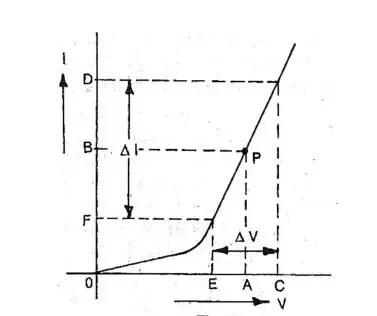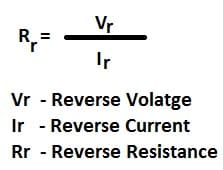# Diode Resistance – Static,Dynamic and Reverse Resistance

The property of the material which offers opposition to flow of electrons or electric current is known as resistance. A p-n junction diode allow current when it is forward biased and blocks the current when it is in reverse biased. However, the diode does not allow the current completely under forward biased and does not block the current in the reversed bias. Ideally, the diode must have zero resistance in forward biasing and infinite resistance in reverse biasing.The depletion layer in p-n junction diode offers resistance to flow of electrons. The resistance offered by diode under forward biased depends on the width of the depletion layer. When forward biased is applied the width of the depletion layer gets decreased. However, the depletion layer can’t be completely eradicated. The thin layer of depletion layer is always exist. The resistance offered by this thin layer of depletion region under forward biased state is called forward resistance of the diode.

When the p-n junction diode is reverse biased the width of the depletion region increases and the charge carriers are blocked by the depletion layer. The resistance of the depletion layer is large because of more width of depletion layer. Under reverse biasing, the diode offers very large resistance to the electric current. This resistance is called the reverse resistance.

In reverse biased, only small amount of electric current due to minority carrier flows through the diode. Thus, the resistance of the diode must be infinite in reverse biased but, practically it does not have infinite resistance because of the current flowing through the depletion layer due to minority charge carriers.

There are two types of resistance of p-n junction diode.

1. Forward Resistance of diode
2. Reverse Resistance of diode

Forward Resistance of Diode
The resistance of the forward biased diode is called forward diode resistance. The forward resistance can be further grouped into two category.

1. Static Resistance or DC resistance
2. Dynamic Resistance or AC resistance

## Static Resistance or DC Forward Resistance of Diode

When DC is fed to diode, the current flows in one direction. The resistance offered by the diode is called the DC resistance.

### Solved Example on Forward Resistance of diode

Calculate the DC resistance of the diode of the following V-I curve.

a) ID = 2 mA

b) ID = 20 mA
c) VD = -10 Volts

The forward resistance of the diode decrease with increase in the forward biasing voltage.

## Dynamic Resistance or AC Forward Resistance of Diode

The resistance offered by the diode when AC is applied to the diode is called AC resistance or dynamic resistance. The current flows in both the direction when AC voltage is applied.The V-I curve of p-n junction diode as as given below.The ratio of change in voltage to change in current represent dynamic resistance of the diode. It is denoted by rac.

## Reverse Resistance of Diode

When reverse bias is applied to p-n junction diode, the width of the depletion layer increase and it offers higher resistance to the flow of charge carriers.

The reverse resistance of p-n diode is in order of mega ohms. The reverse resistance is very large compared to the forward resistance of the diode.

The static reverse resistance of the diode can be calculated by following mathematical expression.

### Formula of Reverse DC Resistance of DiodeThe dynamic reverse resistance of the diode is as follows.